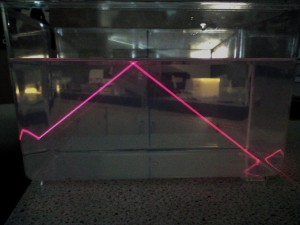## ray diagram (>2f) ipod version

Here is the ipod video version of the ray diagram tutorial for objects more than 2 focal lengths from the lens.

## ray diagram – object further than 2f

This post is for Intermediate 2 only.

The final situation we need to consider is when the object is located more than two focal lengths away from the convex lens.  The diagram is similar to the previous examples but needs additional points marked on the optic axis.

## ray diagram – object closer than 1f

This post is for Standard Grade and Intermediate 2.

Here we consider an object closer than one focal length.  You will see that it is not possible to obtain a real image when the object is this close to the lens.  On a ray diagram, a real image is one that is found on the other side of the lens from the object.

Real images can always be displayed on a screen – a projector in the cinema or classroom produces a real image.  If you are doing an experiment, you can check to see if an image is real using a piece of paper.  Move your sheet of paper closer to and further from the lens – if you can’t get an image to form on the paper then the image must be virtual.  When we look at an object up close through a magnifying glass, we see a virtual image.

## how to draw a ray diagram

If you are sitting Credit Standard Grade or Intermediate 2 you should be able to draw a ray diagram for a convex lens.

At Standard Grade you must be able to draw this diagram for a magnifying glass.  A video for this will be posted shortly.

Intermediate 2 candidates may be asked to draw the diagram for an object placed

• closer than one focal length
• between one and two focal lengths
• more than two focal lengths

from the convex lens.  I will post a video showing each of these three situations.

Let’s start with an introduction to drawing ray diagrams.  This video looks at an object between one and two focal lengths from the lens.  It will show you how to draw the diagram and explain the terms we use to describe the image.

Disclaimer: No rabbits were harmed in the making of this video.

## total internal reflection

By coincidence (pardon the pun!), both Int2 and S3 have reached the stage of investigating total internal reflection at the same time.  Here is a photo showing total internal reflection of a laser beam in a tank of water.I’ve put together a short video showing total internal reflection in a semicircular block and a perspex model of an optical fibre.

## Int2 HW – force momentum energy

Here is your homework exercise on forces, momentum and energy.  Please submit your solutions by Thursday 3rd December.

## Int2 – kinetic energy worked examples

Try working through these kinetic energy problems for yourself.  Ignore any reference to Credit/General, I used these files for Standard Grade as well as Intermediate 2 Physics.